Project Management Central

How can we find regression between an independent variable and a dependent variable measured on nominal/ordinal scale?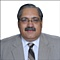Network:84763Here is a research question confusing a lot many scholars. In general, I do not think we can use regression equation in this case. I do understand that there may be a remote possibility of doing so, but that is not the common. We can not use least square regression line to establish the relationship between independent and dependent variables measured on nominal/ordinal scale (likert scale).

What is your take on this?
Sort By: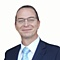Network:7633Hi Suhail, It is a hard talk subject to be answered here in few lines and without charts to display, Let's try to break down the question into definitions first:
The two main variables in an experiment are the independent and dependent variable.

An independent variable is the variable that is changed or controlled in a project to test the effects on the dependent variable.

A dependent variable is the variable being tested and measured in a project.

The dependent variable is 'dependent' on the independent variable. As the experimenter changes the independent variable, the effect on the dependent variable is observed and recorded.

Nominal variables are used to “name,” or label a series of values. Ordinal scales provide good information about the order of choices, such as in a customer satisfaction survey.

The regression of variables can be identified and calculated using different methods :

you have used the word of "Regression" so I will assume that you are looking at Regression Analysis:

Simple Linear Regression
Simple linear regression is a technique that is appropriate to understand the association between one independent (or predictor) variable and one continuous dependent (or outcome) variable.
When there is a single continuous dependent variable and a single independent variable, the analysis is called a simple linear regression analysis . This analysis assumes that there is a linear association between the two variables. (If a different relationship is hypothesized, such as a curvilinear or exponential relationship, alternative regression analyses are performed.

So, in this case, your chart will have two axis X and Y

We could use simple linear regression analysis to estimate the equation of the line that best describes the association between the independent variable and the dependent variable. The simple linear regression equation is as follows:

Y'= B0 +B1X

where Y is the predicted or expected value of the outcome, X is the predictor, b0 is the estimated Y-intercept, and b1 is the estimated slope. The Y-intercept and slope are estimated from the sample data, and they are the values that minimize the sum of the squared differences between the observed and the predicted values of the outcome, i.e., the estimates minimize:
Total of (y-y") power 2

These differences between observed and predicted values of the outcome are called residuals. The estimates of the Y-intercept and slope minimize the sum of the squared residuals and are called the least squares estimates.

Residuals

Conceptually, if the values of X provided a perfect prediction of Y then the sum of the squared differences between observed and predicted values of Y would be 0. That would mean that variability in Y could be completely explained by differences in X. However, if the differences between observed and predicted values are not 0, then we are unable to entirely account for differences in Y based on X, then there are residual errors in the prediction. The residual error could result from inaccurate measurements of X or Y, or there could be other variables besides X that affect the value of Y.
...
1 reply by Suhail Iqbal
May 13, 2018 12:45 PM
Suhail Iqbal
...
Well explained Kevin, but my question remains, can this regression be found for ordinal or nominal scale, because they cannot be measured but only counted. Can we use regression method for attribute (variable characteristic counted not measured)?Network:7633I had to add "residuals" because it is part of the regression resultsNetwork:1624Suhail -

Isn't this a case for multinomial logistic regression?

Kiron
...
1 reply by Suhail Iqbal
May 13, 2018 12:50 PM
Suhail Iqbal
...
Yes Kiron, in that case it would have two independent variables. See if all my variables (independent and dependent) are on ordinal/nominal scale, how could we apply regression on that. With the exception of some specific cases, we cannot apply regression on everything, we may have to apply associations at times. So what do you say?Network:84763May 10, 2018 8:57 PM
Replying to Kevin Drake
...
Hi Suhail, It is a hard talk subject to be answered here in few lines and without charts to display, Let's try to break down the question into definitions first:
The two main variables in an experiment are the independent and dependent variable.

An independent variable is the variable that is changed or controlled in a project to test the effects on the dependent variable.

A dependent variable is the variable being tested and measured in a project.

The dependent variable is 'dependent' on the independent variable. As the experimenter changes the independent variable, the effect on the dependent variable is observed and recorded.

Nominal variables are used to “name,” or label a series of values. Ordinal scales provide good information about the order of choices, such as in a customer satisfaction survey.

The regression of variables can be identified and calculated using different methods :

you have used the word of "Regression" so I will assume that you are looking at Regression Analysis:

Simple Linear Regression
Simple linear regression is a technique that is appropriate to understand the association between one independent (or predictor) variable and one continuous dependent (or outcome) variable.
When there is a single continuous dependent variable and a single independent variable, the analysis is called a simple linear regression analysis . This analysis assumes that there is a linear association between the two variables. (If a different relationship is hypothesized, such as a curvilinear or exponential relationship, alternative regression analyses are performed.

So, in this case, your chart will have two axis X and Y

We could use simple linear regression analysis to estimate the equation of the line that best describes the association between the independent variable and the dependent variable. The simple linear regression equation is as follows:

Y'= B0 +B1X

where Y is the predicted or expected value of the outcome, X is the predictor, b0 is the estimated Y-intercept, and b1 is the estimated slope. The Y-intercept and slope are estimated from the sample data, and they are the values that minimize the sum of the squared differences between the observed and the predicted values of the outcome, i.e., the estimates minimize:
Total of (y-y") power 2

These differences between observed and predicted values of the outcome are called residuals. The estimates of the Y-intercept and slope minimize the sum of the squared residuals and are called the least squares estimates.

Residuals

Conceptually, if the values of X provided a perfect prediction of Y then the sum of the squared differences between observed and predicted values of Y would be 0. That would mean that variability in Y could be completely explained by differences in X. However, if the differences between observed and predicted values are not 0, then we are unable to entirely account for differences in Y based on X, then there are residual errors in the prediction. The residual error could result from inaccurate measurements of X or Y, or there could be other variables besides X that affect the value of Y.
Well explained Kevin, but my question remains, can this regression be found for ordinal or nominal scale, because they cannot be measured but only counted. Can we use regression method for attribute (variable characteristic counted not measured)?Network:84763May 11, 2018 8:37 AM
Replying to Kiron Bondale
...
Suhail -

Isn't this a case for multinomial logistic regression?

Kiron
Yes Kiron, in that case it would have two independent variables. See if all my variables (independent and dependent) are on ordinal/nominal scale, how could we apply regression on that. With the exception of some specific cases, we cannot apply regression on everything, we may have to apply associations at times. So what do you say?Network:1624Suhail -

Ordinal logistic regression is likely what you need then - here's a couple of interesting links:

https://www.st-andrews.ac.uk/media/capod/s...nalexampleR.pdf

https://www.researchgate.net/post/Can_we_u...ession_analysis

Kiron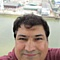Network:2435Kevin, well done on briefing the regression, do you know it is my favorite indicator but it is different from what looks like on Kiron reference website
...
1 reply by Kevin Drake
Jul 28, 2018 10:00 PM
Kevin Drake
...
Good manNetwork:7633Jul 28, 2018 7:58 PM
...
Kevin, well done on briefing the regression, do you know it is my favorite indicator but it is different from what looks like on Kiron reference website
Good man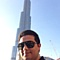Network:657Kiron you are right!!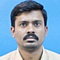Network:7255Very elaborate explanation by Kevin. Appreciate your time & patience